• 蛮力法蛮力法概述蛮力法的基本应用直接采用蛮力法的一般格式例1例2 蛮力法概述 蛮力法是一种简单直接地解决问题的方法，通常直接基于问题的描述和所涉及的概念定义，找出所有可能的解。然后选择其中的一种或多种解，...
蛮力法蛮力法概述蛮力法的基本应用直接采用蛮力法的一般格式例1例2
蛮力法概述
蛮力法是一种简单直接地解决问题的方法，通常直接基于问题的描述和所涉及的概念定义，找出所有可能的解。然后选择其中的一种或多种解，若该解不可行则试探下一种可能的解。
使用蛮力法通常有如下几种情况：
搜索所有的解空间：问题的解存在于规模不大的解空间中。
搜索所有的路径：这类问题中不同的路径对应不同的解。
直接计算：按照基于问题的描述和所涉及的概念定义，直接进行计算。往往是一些简单的题，不需要算法技巧的。
模拟和仿真：按照求解问题的要求直接模拟或仿真即可。
蛮力法的基本应用
直接采用蛮力法的一般格式
在直接采用蛮力法设计算法中，主要是使用循环语句和选择语句，循环语句用于穷举所有可能的情况，而选择语句判定当前的条件是否为所求的解。
例1
编写一个程序，输出2～1000之间的所有完全数。所谓完全数，是指这样的数，该数的各因子（除该数本身外）之和正好等于该数本身，例如：
6=1+2+3
28=1+2+4+7+14
先考虑对于一个整数m，如何判断它是否为完全数。
从数学知识可知：一个数m的除该数本身外的所有因子都在1～m/2之间。算法中要取得因子之和，只要在1～m/2之间找到所有整除m的数，将其累加起来即可。如果累加和与m本身相等，则表示m是一个完全数，可以将m输出。
void main()
{  int m,i,s;
for (m=2;m<=1000;m++)
{  s=0;
for (i=1;i<=m/2;i++)
if (m%i==0) s+=i;	//i是m的一个因子
if (m==s)
printf("%d ",m);
}
printf("\n");
}


例2
在象棋算式里，不同的棋子代表不同的数，有以下算式，设计一个算法求这些棋子各代表哪些数字。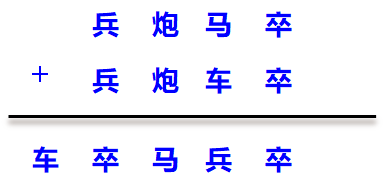采用蛮力法时，设兵、炮、马、卒和车的取值分别为a、b、c、d、e。则有：
a、b、c、d、e的取值范围为0～9且均不相等
（a==b || a==c || a==d || a==e || b==c || b==d || b==e || c==d || c==e || d==e）

该表达式不成立
设：
m=a×1000+b×100+c×10+d
n=a×1000+b×100+e×10+d
s=e×10000+d×1000+c×100+a×10+d
则有：m+n==s
void fun()
{  int a,b,c,d,e,m,n,s;
for (a=1;a<=9;a++)
for (b=0;b<=9;b++)
for (c=0;c<=9;c++)
for (d=0;d<=9;d++)
for (e=0;e<=9;e++)
if (a==b || a==c || a==d ||
a==e || b==c || b==d ||
b==e || c==d || c==e ||  d==e)
continue;
else
{  m=a*1000+b*100+c*10+d;
n=a*1000+b*100+e*10+d;
s=e*10000+d*1000+c*100+a*10+d;
if (m+n==s)
printf("兵:%d 炮:%d 马:%d卒:%d 车:%d\n",
a,b,c,d,e);
}
}




展开全文算法 c++
• 蛮力法蛮力法蛮力法蛮力法蛮力法蛮力法蛮力法蛮力法蛮力法蛮力法蛮力法
• 之前写了一篇Python蛮力法解决凸包问题并用matplotlib实现可视化，最后也给出了同样是在1000个点的情况下蛮力法和分治法的差距有多大(蛮力法1154秒，分治法0.125秒…) 先解释一下为什么吧： 因为蛮力法的重点在于...
• 蛮力法的详细介绍及其描述，是你可以全新的认识蛮力法
• 蛮力法求一些经典算法，背包问题，凸包问题的蛮力算法等等选择排序
• 本资源包含“基于蛮力法（DFS）解决TSP问题”的相关代码以及TSP的城市数据。 本资源包含“基于蛮力法（DFS）解决TSP问题”的相关代码以及TSP的城市数据。
• 蛮力法新手可看一下，超级入门代码，看看入门蛮力法，高手绕路
• C++算法设计中的蛮力法，虽然时间效率比较低，但有些问题还必须使用蛮力法来解决。当问题规模较小时，蛮力法设计简单，不失为一个好方法。下面列举几个用蛮力法解决的问题，帮助大家理解蛮力法的设计思想。 **查找...
C++算法设计中的蛮力法，虽然时间效率比较低，但有些问题还必须使用蛮力法来解决。当问题规模较小时，蛮力法设计简单，不失为一个好方法。下面列举几个用蛮力法解决的问题，帮助大家理解蛮力法的设计思想。
**查找问题 **
int Find(int a[],int n,int k){
int i=n;
a=k;
while(a[i]!=k)
i--;
return i;
}

测试：
	clock_t start,finish;
double totaltime;
start=clock();
int a[]={0,1,2,3,4,5,6,7,8,9};
cout<<Find(a,10,6);
finish=clock();
totaltime=(double)(finish-start)/CLOCKS_PER_SEC;
cout<<"\n此程序的运行时间为"<<totaltime<<"秒！"<<endl;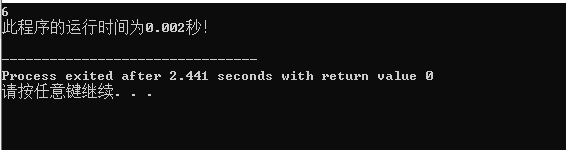生成子集问题
void CreateSet(int n){
int i,s=1;
for(i=0;i<n;i++){
s*=2;
}
for(i=0;i<s;i++){
cout<<i<<" ";
printf("%o",i);//8进制  %x 16进制
cout<<endl;
}
}

测试;
    CreateSet(5);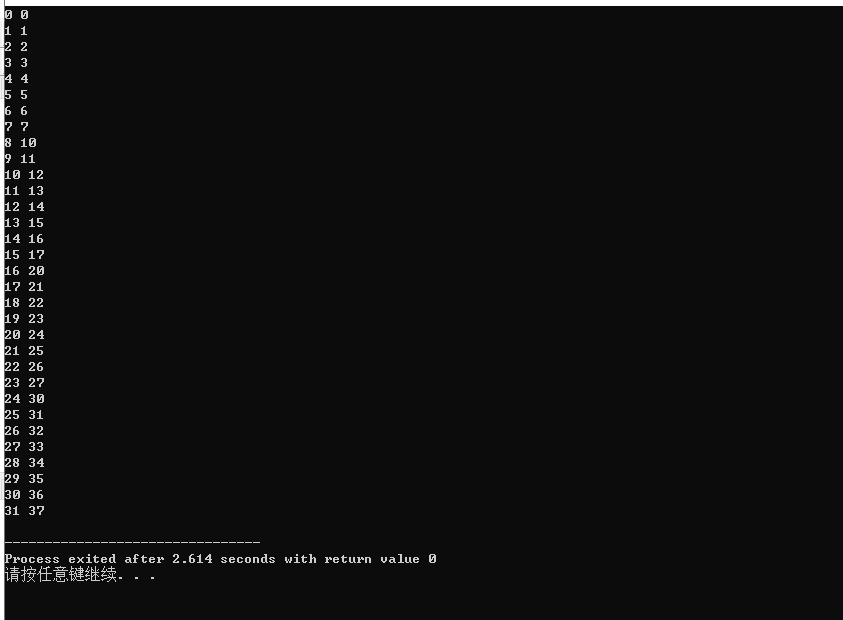凸包问题
void ConvexHull(int x[],int y[],int n){
int i,j,k,sign1,sign2;
int A,B,C;
for(i=0;i<n-1;i++){
for(j=i+1;j<n;j++){
sign1=0;sign2=0;
A=y[i]-y[j];
B=x[j]-x[i];
C=x[i]*y[j]-x[j]*y[i];
for(k=0;k<n;k++){
if(k!=i&&k!=j){
if(A*x[k]+B*y[k]+C>0)
sign1=1;
else
sign2=1;
if(sign1==sign2) break;
}
}
if(k==n){
cout<<"("<<i<<","<<j<<")"<<endl;
}
}
}
}

测试：
    int x[]={0,1,2,2,3};
int y[]={0,2,1,6,0};
ConvexHull(x,y,5);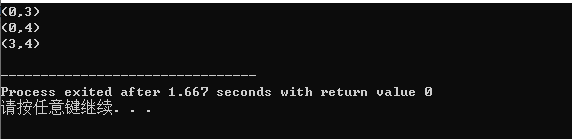展开全文凸包问题 C++
• 蛮力法概念： 4.2.1 直接采用蛮力法的一般格式
蛮力法概念：4.2.1 直接采用蛮力法的一般格式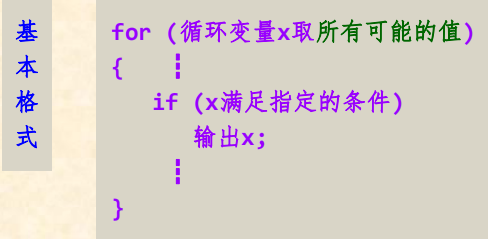展开全文机器学习
• 此程序用蛮力法求解旅行商问题，输入城市数目得出最优解，将运算时间存储到外部文件，精确到毫秒。
• 算法设计与分析之二 蛮力法 目录 1.蛮力法的设计思想 2.蛮力法优点 3. 冒泡排序分析 4. 选择排序分析 5. 蛮力法中冒泡排序与选择排序的时间空间复杂度分析 6. 蛮力法C语言实现 7. 算法稳定性的问题 8. 百钱买百鸡的...
算法设计与分析之二  蛮力法
目录

1.蛮力法的设计思想
2.蛮力法优点
3. 冒泡排序分析
4. 选择排序分析
5. 蛮力法中冒泡排序与选择排序的时间空间复杂度分析
6. 蛮力法C语言实现
7. 算法稳定性的问题
8. 百钱买百鸡的问题
9. 补充：鸡尾酒排序法
10.蛮力法的思考

作为算法设计技术中最简单的一种设计策略，蛮力法从最先开始接触编程语言时就一直伴随着我们。
1. 蛮力法的设计思想
课本上的定义：蛮力法是指一种简单直接

1.蛮力法又称为枚举法，穷举法，暴力法。
2.蛮力法是指采用遍历（扫描）技术，即采用一定的策略将待求解问题的所有元素依次处理一次，从而找出问题的解。依次处理所有元素是蛮力法的关键，为了避免陷入重复试探，应保证处理过的元素不再被处理。

蛮力法解决问题的方法

根据问题中的条件将可能的情况一一列举出来，逐一尝试从中找出满足问题条件的解。但有时一一列举出的情况数目很大，如果超过了我们所能忍受的范围，则需要进一步考虑，排除一些明显不合理的情况，尽可能减少问题可能解的列举数目。

蛮力法解决问题的算法设计：

1）找出枚举范围：分析问题所涉及的各种情况。
2）找出约束条件：分析问题的解需要满足的条件，并用逻辑表达式表示。

2.蛮力法优点

逻辑清晰，编写程序简洁
对于一些重要的问题（比如：排序、查找、矩阵乘法和字符串匹配），可以产生一些合理的算法
解决问题的实例很少时，可以花费较少的代价
可以解决一些小规模的问题（使用优化的算法没有必要，而且某些优化算法本身较复杂)
可以作为其他高效算法的衡量标准

3. 冒泡排序分析（稳定性排序方法）
第i趟排序对序列的前n-i+1个元素从第一个元素开始依次作如下操作:相邻的两个元素比较大小，若前者大于后者,则两个元素交换位置，否则不交换位置。该n-i+1个元素中最大值元素移到该n-i+1个元素的最后。冒泡排序方法比较适合于参加排序的序列的原始状态基本有序的情况。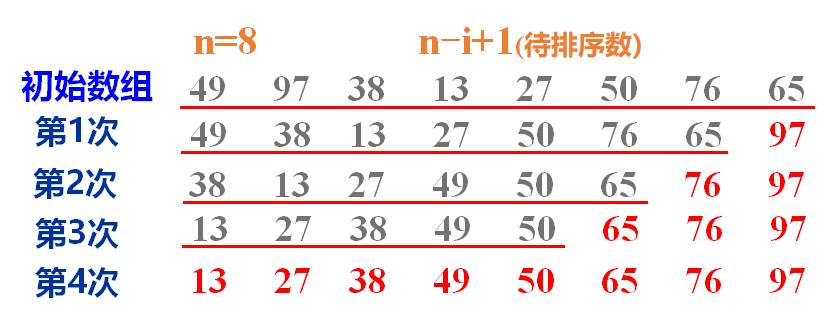4. 选择排序分析（非稳定性排序方法）
选择排序开始的时候，扫描整个序列，找到整个序列的最小记录和序列中的第一个记录交换，从而将最小记录放到它在有序区的最终位置上，然后再从第二个记录开始扫描序列，找到n-1个序列中的最小记录，再和第二个记录交换位置。一般地，第i趟排序从第i个记录开始扫描序列，在n-i+1（1≤i≤n-1）个记录中找到关键码最小的记录，并和第i个记录交换作为有序序列的第i个记录。
每一趟排序从序列中未排序的元素中选择一个值最小的元素，将其置于没有排好序的元素的最前面。已排好序的元素不必交换。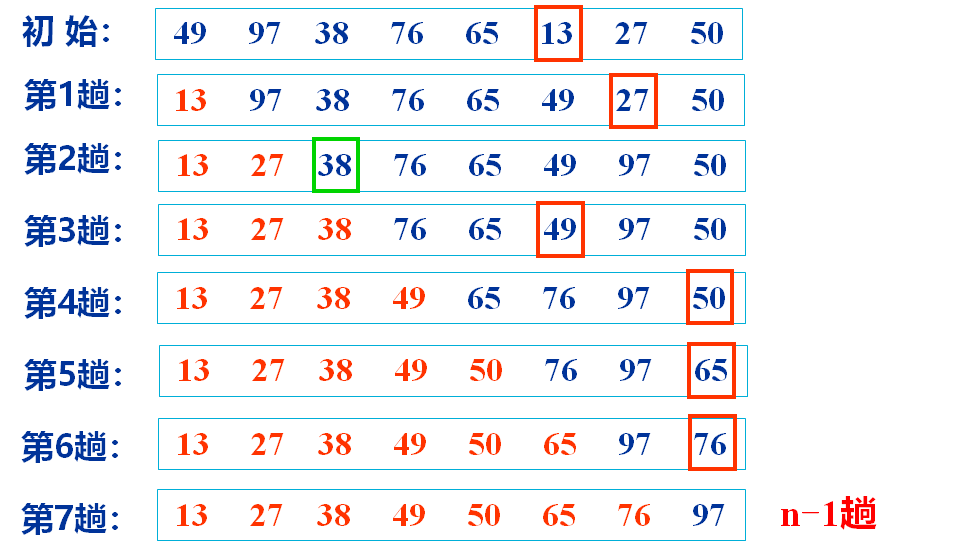5. 蛮力法中冒泡排序与选择排序的时间空间复杂度分析
1.选择排序：

平均时间复杂度o(n^2 )
最好时间复杂度o(n^2 )
最坏时间复杂度o(n^2 )
空间复杂度o(1)

2.冒泡排序：

平均时间复杂度o(n^2 )
最好时间复杂度o(n)
最坏时间复杂度o(n^2 )
空间复杂度o(1)

6.1蛮力法中冒泡排序C语言实现
#include <stdio.h>
void maopaopaixu(int s[],int n);
void main()
{
int s;
int i;
int n;
printf("请输入要输入的个数：");
scanf("%d",&n);
printf("请输入要排序的序列：\n");
for (i = 0; i < n; i++)
{
scanf("%d",&s[i]);
}
maopaopaixu(s,n);
printf("排序后的序列：\n");
for (i = 0; i < n; i++)
{
printf("%d\t",s[i]);
}
}
void maopaopaixu(int s[],int n)
{
int i, j;
int temp;
for(i = 0; i < n; i++)
{
for (j = 0; j < n-1; j++)
{
if(s[i] < s[j])
{
temp = s[i];
s[i] = s[j];
s[j] = temp;
}
}
}
}

6.2蛮力法中选择排序C语言实现
#include <stdio.h>
void suanzepaixu(int s[],int n);
void main()
{
int n;
int i;
int s;
printf("请输入要输入的个数");
scanf("%d",&n);
printf("请输入要排序的数据\n");
for(i = 0; i < n; i++)
{
scanf("%d",&s[i]);
}
suanzepaixu(s,n);
for (i = 0; i < n; i++)
{
printf("%d\t",s[i]);
}
}
void suanzepaixu(int s[],int n)
{
int i,j;
int k;
int temp;
for (i = 0; i < n; i++)
{
k = i;
for(j = i + 1; j < n; j++)
{
if(s[j] < s[k])
{
k = j;
}
}
if(k != i)
{
temp = s[k];
s[k] = s[i];
s[i] = temp;
}
}
}

7.算法稳定性的问题
冒泡排序：

冒泡排序就是把小的元素往前调或者把大的元素往后调。比较是相邻的两个元素比较，交换也发生在这两个元素之间。所以，如果两个元素相等，不用交换；如果两个相等的元素没有相邻，那么即使通过前面的两两交换把两个相邻起来，这时候也不会交换，所以相同元素的前后顺序并没有改变，所以冒泡排序是一种稳定排序算法。

选择排序：

选择排序即扫描整个序列，找到整个序列的最小记录和序列中的第一个记录交换，从而将最小记录放到它在有序区的最终位置上，然后再从第二个记录开始扫描序列，找到n-1个序列中的最小记录，再和第二个记录交换位置。一个序列当如果当前元素比一个元素小，而该小的元素又出现在一个和当前元素相等的元素后面，那么交换后稳定性就被破坏了。因此选择排序是非稳定性的。

8.百钱买百鸡问题
数学家张丘建提出百鸡百钱问题，今有鸡翁一，值钱五，鸡母一，值钱三，鸡雏三，值钱一。百钱买百鸡，问鸡翁，鸡母，鸡雏各几何？翻译后：设鸡翁，鸡母，鸡雏分别为x,y,z，给出一百钱要买百鸡。如果全买公鸡最多买20只，显然x在0—20之间，同理y的取值在0-33之间，所以根据分析，不难用枚举法求出问题的所有符合情况的解。
这是个经典问题，从我们学数学就避不开的问题，用蛮力法实现应该是最不费脑筋的。首先，简单分析一下枚举范围，都买公鸡最多二十只，x的范围0-20，都买母鸡最多30只，y的范围0-30。
#include <stdio.h>
//鸡翁x,鸡母y,鸡雏z
void main()
{
int z = 0;
int x, y;
//鸡翁的数量变化范围
for (x= 0; x < 20; x++)
{
//鸡母的数量变化范围
for (y = 0; y < 33; y++)
{
z = 100 - x - y;
//鸡雏的受制约范围
if (z % 3 == 0 && 5 * x + 3 * y + z / 3 == 100)
{
printf("x = %d,y = %d,z = %d\n",x,z,y);
}
}
}
}

9.鸡尾酒排序

鸡尾酒排序法，又名双向冒泡排序法，算法传统冒泡法的一点改进。但是对于鸡尾酒排序，算法的时间复杂度与空间复杂度并没有改进。
不同的是排序的交换次数。某些情况下鸡尾酒排序比普通冒泡排序的交换次数少。比如{2,3,4,1},鸡尾酒排序只需交换2次，而冒泡排序需要三次。总体上，鸡尾酒排序可以获得比冒泡排序稍好的性能。但是完全逆序时，鸡尾酒排序与冒泡排序的效率都非常差。
鸡尾酒排序的思想就是在从前往后依次循环依靠邻近数据交换实现结果的同时，依次从后往前循环数据交换。前者交换获取未排序最大值，而后者交换获取未排序最小值。实现过程如下图：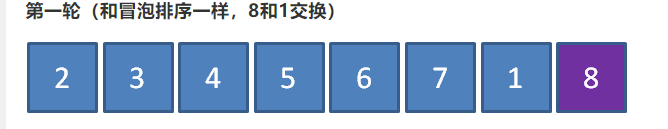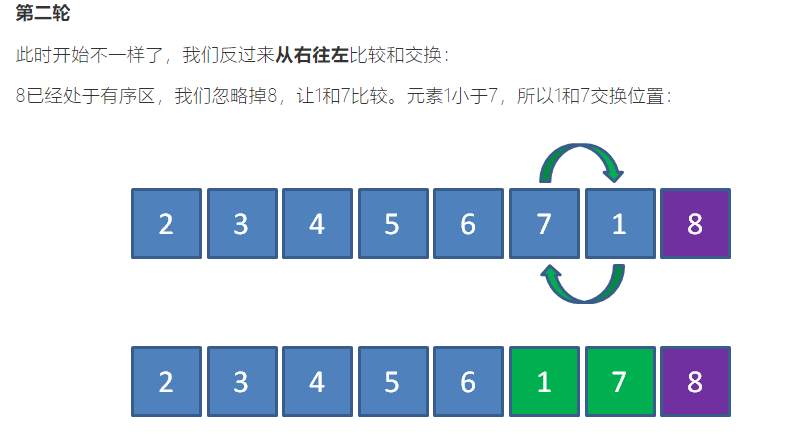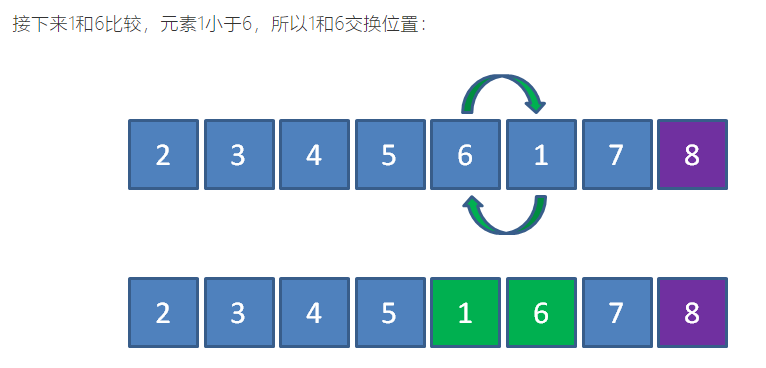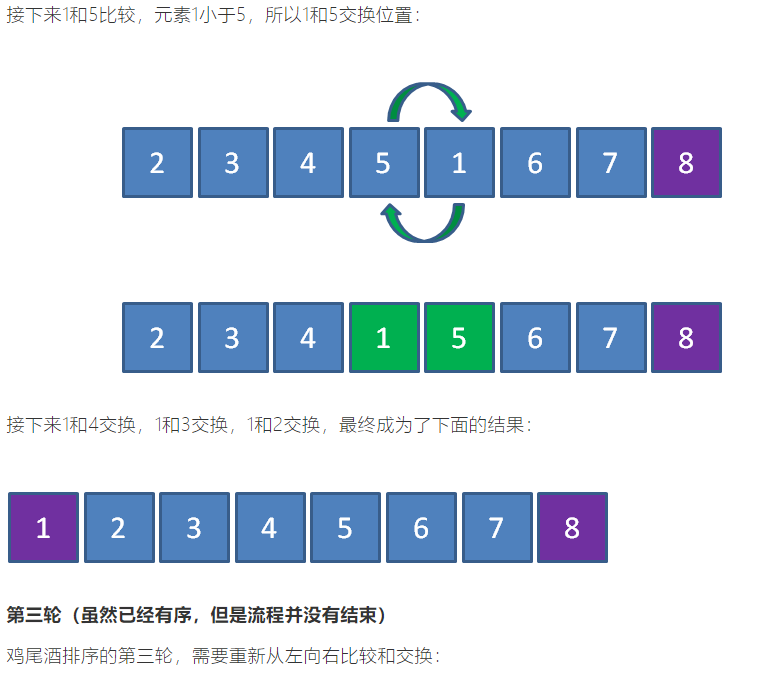python代码
l = [2,3,4,1,90,6]
size = len(l)
sign = 1
for i in range(int(size / 2)):
if sign:
sign = 0
for j in range(i, size - 1 - i):
if l[j] > l[j + 1]:
l[j], l[j + 1] = l[j + 1], l[j]
for k in range(size - 2 - i, i, -1):
if l[k] < l[k - 1]:
l[k], l[k - 1] = l[k - 1], l[k]
sign = 1
else:
break
print(l)

10.蛮力法的思考
用蛮力法设计的算法，一般来说，经过适度的努力后，都可以对算法的第一个版本进行一定程度的改良，改进其时间性能，但只能减少系数，而数量级不会改变。


展开全文C语言
• 蛮力法的主要思想就是用最简单的思路解决问题，一般性能不好，但仍然很重要。 理论上蛮力法可以解决可计算领域的各种问题 蛮力法解决较小规模问题是可接受的，如果设计一个更高效算法代价不值得 蛮力法可以作为时间...后端
• 文章目录算法学习之蛮力法蛮力法的思想蛮力法的基本蛮力法的优点 蛮力法的思想 蛮力法是指采用遍历技术，使用一定的策略将待求解问题的所有元素依次处理一次，其关键为依次处理所有元素，要使已处理过的元素不再被...算法
• 算法01-蛮力法一、蛮力法介绍蛮力法(brute force method，也称为穷举法或枚举法)是一种简单直接地解决问题的方法，常常直接基于问题的描述，所以，蛮力法也是最容易应用的方法。但是，用蛮力法设计的算法时间特性...
• 文章目录蛮力法的定义蛮力法的优缺点蛮力法的设计思想蛮力法的经典使用排序选择排序冒泡排序顺序查找字符串匹配问题最近点对的蛮力算法凸包问题的蛮力算法穷举法NP难问题TSP问题背包问题分配问题 蛮力法的定义 蛮力......